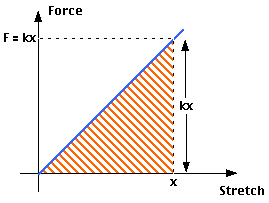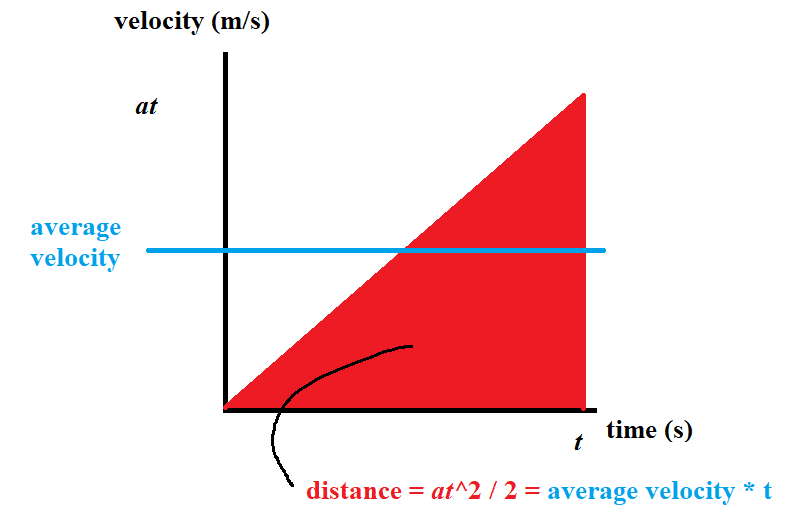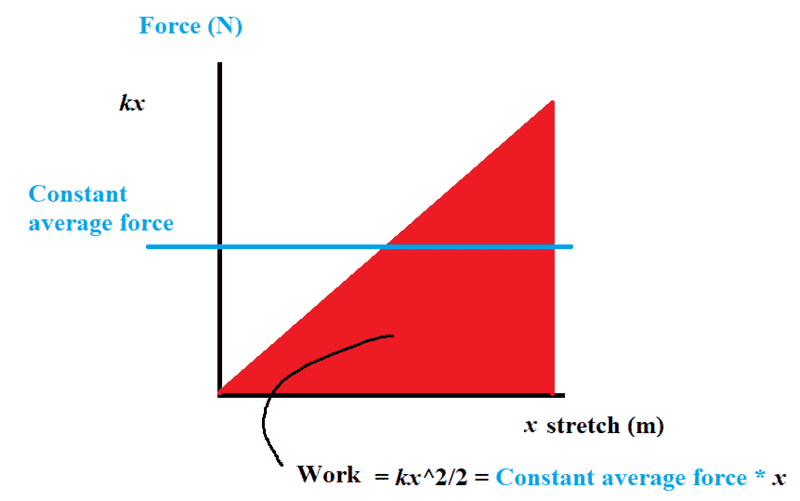# Questions about a spring and its principle

• I
• Sangari Indiraj
In summary, there is confusion about when to use the formula 1/2ke2 for calculating the work done on a spring. The energy conservation theorem and the graphical method of finding work done give different answers, but this is because they are solving different problems. It is also recommended to have a basic understanding of calculus for better comprehension of these concepts.

#### Sangari Indiraj

Summary: I am confused in when to use 1/2 e squared k

we studied in class that normally, the extension of the spring is directly proportional to the tension applied
and we did this question :
what is the work that has to be done for a spring to have an extension of length e
as the area of the force extension graph gives the work done, we get the formula as 1/2ke2.
but in the next question :
A weightless spring is attached to a ceiling and a weight of 20 N is applied. Here the spring extend for 5cm. Calculate the spring constant.
solution1:
f= ek
k= 20 / 0.05
=400 N m-1
solution 2
according to the energy conservation theorum,
mgh = 1/2 e2 k
20* 0.05=1/2 * 0.05 * 0.05 *k
k=800 N m-1

why am i getting two different answers? It will help if anyone explains. Also please don't use derivatives. I cannot understand them.

•PeroK
Sangari Indiraj said:
Summary: I am confused in when to use 1/2 e squared k

we studied in class that normally, the extension of the spring is directly proportional to the tension applied
and we did this question :
what is the work that has to be done for a spring to have an extension of length e
as the area of the force extension graph gives the work done, we get the formula as 1/2ke2.
but in the next question :
A weightless spring is attached to a ceiling and a weight of 20 N is applied. Here the spring extend for 5cm. Calculate the spring constant.
solution1:
f= ek
k= 20 / 0.05
=400 N m-1
solution 2
according to the energy conservation theorum,
mgh = 1/2 e2 k
20* 0.05=1/2 * 0.05 * 0.05 *k
k=800 N m-1

why am i getting two different answers? It will help if anyone explains. Also please don't use derivatives. I cannot understand them.
Your energy conservation equation implies that we are going to drop the mass. So the spring length e is going to be longer than its equilibrium length. When the mass is just hanging then the forces cancel out and you have the equilibrium length of the spring. The two problems are different.

-Dan

•PeroK
... if you drop the mass, then it will oscillate under simple harmonic motion. The mass will in fact have its maximum speed, hence maximum KE, at the equilibrium point. When it has zero velocity, it will have fallen twice as far.

If you lower the mass into the equilibrium position, then you have an additional external force doing negative work on the system- in order to stop the mass accelerating under gravity.

•topsquark
Sangari Indiraj said:
Also please don't use derivatives. I cannot understand them.
Perhaps it would be better to spend your time getting to grips with fairly elementary Calculus. You could then have the answer to your question (and many other basic questions), all on a plate.
This link shows an easy graphical method to show where the 'half' factor comes from. This diagram is explained fairly well in the link.Visualise stretching the spring a tiny bit at a time. The force will not change noticeably so the work done will be kx times the small stretch Δx. The total work done will be the sum of all those small stretches, which is represented by the shaded part of the triangle. Area is half base times height.

•malawi_glenn
same graph as above, but imagine there was a constant average force instead stretching the spring x. This average force would be kx/2 and the work done would be kx2/2.
You are probably familiar with this method in finding the distance traveled when you have constant acceleration.

•topsquark
malawi_glenn said:
same graph as above, but imagine there was a constant average force instead stretching the spring x. This average force would be kx/2 and the work done would be kx2/2.
I don't think you actually meant that. The force to keep the spring at x would be kx (not half). If that force were applied over a distance x then the work would be kx2. That's twice as much as if the force is build up gradually.

•Sangari Indiraj and topsquark
malawi_glenn said:
You are probably familiar with this method in finding the distance traveled when you have constant acceleration.
I used to have terrible problems with that until I stopped thinking of a practical car engine. No one stressed the 'constant acceleration' enough to get through my thick skull.

•topsquark and malawi_glenn
sophiecentaur said:
I don't think you actually meant that. The force to keep the spring at x would be kx (not half). If that force were applied over a distance x then the work would be kx2. That's twice as much as if the force is build up gradually.•topsquark
malawi_glenn said:
A picture is worth a thousand words but you described stretching ‘the spring’ by x. Hence the confusion.

•topsquark
sophiecentaur said:
I don't think you actually meant that. The force to keep the spring at x would be kx (not half). If that force were applied over a distance x then the work would be kx2. That's twice as much as if the force is build up gradually.
thankyou this is where am confused.

## 1. What is Hooke's Law and how does it relate to springs?

Hooke's Law states that the force applied to a spring is directly proportional to the extension or compression of the spring, as long as the spring is not stretched beyond its elastic limit. This means that the more force you apply to a spring, the more it will stretch or compress.

## 2. How does the stiffness of a spring affect its behavior?

The stiffness, or spring constant, of a spring determines how much force is required to extend or compress it. A stiffer spring will require more force to stretch or compress compared to a less stiff spring. This affects the behavior of the spring by changing its resistance to deformation.

## 3. What is the relationship between the length of a spring and its force constant?

The force constant, or spring constant, is directly proportional to the length of the spring. This means that as the length of the spring increases, so does the force constant. This relationship is expressed mathematically as F = kx, where F is the force, k is the spring constant, and x is the length of the spring.

## 4. Can the spring constant of a spring change?

Yes, the spring constant of a spring can change. It is affected by factors such as the material and thickness of the spring, as well as any changes in the temperature or environment. For example, a metal spring may become stiffer in colder temperatures.

## 5. How does the mass attached to a spring affect its behavior?

The mass attached to a spring affects its behavior by changing the force required to stretch or compress the spring. A heavier mass will require more force to move the spring compared to a lighter mass. This relationship is expressed mathematically as F = mg + kx, where F is the total force, m is the mass, g is the acceleration due to gravity, k is the spring constant, and x is the displacement of the spring.